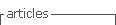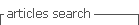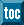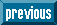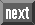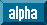## Indicators

•Cited by SciELO
•Access statistics

•Cited by Google
•Similars in SciELO
•Similars in Google

## Print version ISSN 0121-1129

### Rev. Fac. ing. vol.27 no.48 Tunja May/Aug. 2018

#### http://dx.doi.org/10.19053/01211129.v27.n48.2018.8403

Artículos

Mathematical software tools For teaching of complex numbers

Herramientas de software matemático para la enseñanza de números complejos

Ferramentas de software matemático para o ensino de números complexos

Brayan Esguerra-Prieto*  , Natalia González-Garzón**  , Alberto Acosta-López***

* Universidad Distrital Francisco José de Caldas (Bogotá-Distrito Capital, Colombia). baesguerrap@correo.udistrital.edu.co.

** Universidad Distrital Francisco José de Caldas (Bogotá-Distrito Capital, Colombia). nagonzalezg@correo.udistrital.edu.co.

*** M. Sc. Universidad Distrital Francisco José de Caldas (Bogotá-Distrito Capital, Colombia). aacosta@udistrital.edu.co.

Abstract

This article proposes a method for teaching complex numbers and their corresponding operations using mathematical software tools. Initially, the document introduces MATLAB, a paid software, and GeoGebra, a free software. Subsequently, the paper explains the procedure to define the representations of the basic (addition, difference, multiplication and division) and complementary (module and conjugate, roots and polar form) operations of the numerical system of complex numbers, using the two tools and a series of proposed exercises. Finally, the paper highlights the evidenced qualities during the development of the exercises and their transcendence in teaching and learning.

Key words: computer-aided instruction; electronic learning; interactive systems; mathematics education; software tools

Resumen

El artículo propone un método para enseñar los números complejos y sus correspondientes operaciones mediante herramientas de software matemático. Inicialmente se presentan las herramientas MATLAB, cuya licencia es de pago, y GeoGebra, que es un software libre. En la segunda parte se explica el procedimiento para definir las representaciones de las operaciones básicas (adición, diferencia, multiplicación y división) y complementarias (módulo y conjugado, raíces, forma polar) del sistema numérico de los números complejos, usando cada una de las dos herramientas y utilizando una serie de ejercicios propuestos. Finalmente, se diferencian las cualidades evidenciadas durante el desarrollo de los ejercicios y su trascendencia en la enseñanza y aprendizaje.

Palabras-clave: aprendizaje por computadoras; enseñanza de las matemáticas; enseñanza virtual; herramientas de software; sistemas interactivos

Resumo

O artigo propõe um método para ensinar os números complexos e suas correspondentes operações mediante ferramentas de software matemático. Inicialmente apresentam-se as ferramentas MATLAB, cuja licença é paga, e GeoGebra, que é um software livre. Na segunda parte explica-se o procedimento para definir as representações das operações básicas (adição, subtração, multiplicação e divisão) e complementárias (módulo e conjugado, raízes, forma polar) do sistema numérico dos números complexos, usando cada uma das duas ferramentas e utilizando uma série de exercícios propostos. Finalmente, diferenciam-se as qualidades evidenciadas durante o desenvolvimento dos exercícios e sua transcendência no ensino e aprendizagem.

Palavras-Chave: aprendizagem por computadores; ensino de matemática; ensino virtual; ferramentas de software; sistemas interativos

I. INTRODUCTION

Today, Information and Communication Technologies (ICT) provide multiple tools for learning mathematics that are necessary for learning complex numbers. Complex numbers have applications in different fields, such as engineering (description of electrical circuits and electromagnetic waves), physics, chemistry, biology, mathematics and statistics, among others. Several concepts that include complex numbers require visual comprehension that is difficult to understand in a guide book or in a lecture. Using interactive tools for understanding this topic allows the student to comprehend the topics better.

All new technologies allow an increasingly important development in each of the daily activities, use them as an important tool to acquire or develop knowledge makes the application of theoretical concepts are simpler, and ICTs become an entertaining way to learn. It is essential that in order to obtain greater interaction with the new environments for the learning of complex numbers, we have previous knowledge on the subject and on the use of the tools to be used, in this case MATLAB and GeoGebra.

MATLAB is a mathematical software tool that uses its own programming language; it was created in 1984 in Albuquerque, Nuevo México, by a mathematician and programmer named Cleve Moler, and its name comes from the abbreviation of the words MATrix and LABoratory. The latest stable version is the R2018a, released in March of 2018. GeoGebra is a mathematical software application specialized in geometry, algebra and calculus. It is a free software, which makes it accessible to more people, and presents a friendly graphic environment simpler for the user. It was created in 2002 by Markus Hohenwarter from the University of Salzburgo, Austria, as his master's thesis, and its name comes from "Geo" from Geometry and "Gebra" from the Fundamental Theorem of Algebra. The most recent version is 6.0.465.0, launched on May of 2018.

Like this representation, it is possible to graph the results by means of the following form :

II. METHODOLOGY

The methodology used during the development of the research is presented below. The potential of interactive systems in mathematics education and the tools proposed for a better understanding of complex numbers are discussed.

Learning complex numbers can often be a complex task for students and the general public , . However, technology allows developing learning in an interactive way . The irruption of information and communication technologies (ICT) "as an educational tool represents a turning point in the way in which learning [...], breaking with rigid schemes and traditional teaching has been done, to adopt other ways of building, managing and transmitting knowledge" .

Technological tools like MATLAB, GeoGebra , SciLab, Wolfram Alpha, and others evolve every day to provide better content, power and efficiency at the time of plotting or manipulating mathematical content . Among the many tools that can be used, we have chosen MATLAB and GeoGebra, which will show the process of developing basic and some complementary operations with complex numbers, each one with its advantages and differences, to provide the user with information to choose the preferred tool.

III. RESULTS

Below are the results obtained after the application of some examples using the proposed tools: MATLAB and GeoGebra.

A. Complex numbers in MATLAB

The environment of MATLAB is designed to be used directly through commands. In addition, it is possible to graphically display the results . We can create a complex number in MATLAB in the following ways :

>>plot(z1,’og’,’MarkerSize’,7,’ Marker Face Color’,’g’); text(real(z1)+0.08,imag(z1), ‘12 + 7i’)

>> grid on

>> title (' Representation of an Imaginary number)

• plot: Is the command that allows to graph or to represent some numerical expression.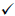MarkerSize - Specifies the marker pixel sizeMarkerFaceColor - Determines the fill color that will have the figure representing the point to be plotted

• text: Allows you to assign a text to the point that was plotted, in this case, drawing the coordinates of the number plotted to establish its correct position.

• grid on: Draws a grid on the graph to distinguish the position of the graphed points more easily.

• title: Allows you to assign a title to the graph.

• figure: Receives as a parameter a number that will assign an order to the drawn figures.

• hold on: Allows drawing some of the points in a fixed way, without the possibility of being drawn when drawing another new point or updating the graph.

Operations between complexes: As an example, below, we will show operations established for complex numbers, such as basic operations and module, conjugate, complex number roots, and polar form .

Addition and Subtraction: It starts by defining the numbers that we are going to use for each operation, in this case, the sums will be z1 and z2, and the variable z3 will store the result of the sum .

>> z1 = -4+3i;

>> z2 = 6 - 10i;

>> z3 = z1+z2

z3 = 2.0000 - 7.0000i

Graph: In this process, the commands explained above are used to plot all the points of the complex numbers defined and their corresponding result (Fig. 1). Once we entered the following commands in the MATLAB command window, we obtained the graph on the left (Fig 1.)

>> grid on

>>plot(z1,’ob’,’MarkerSize’,7,

’MarkerFaceColor’, ’b’); text(real(z1) + 0.08,

imag(z1), ‘-4+3i’)

>> hold on

>>plot(z2,’ob’,’MarkerSize’,

7,’MarkerFaceColor’, ’b’);

text(real(z2)+0.08,imag(z2),’6-10i’)

>> grid on

>>plot(z3,’or’,’MarkerSize’,

7,’MarkerFaceColor’, ’r’);

text(real(z3)+0.08,imag(z3),’2-7i’)

>> hold on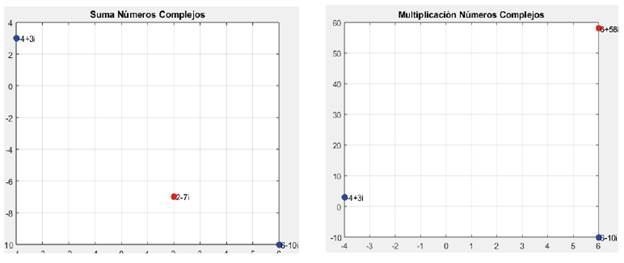FIG. 1 Complex Numbers Addition and Multiplication in MATLAB (the sums are shown in blue and the result is red)

The procedure for subtraction is the same.

Multiplication and division: The variables z1 and z2 are multiplied and the result is stored in the z5 variable

>> z5 = z1*z2

z5 =

6.0000 +58.0000i

Graph: To produce the graph, we introduced the following commands and obtained the graph on the right (Fig. 1). The same procedure is performed for division.

>> grid on

>>plot(z1,’ob’,’MarkerSize’,

7,’MarkerFaceColor’,’b’);

text(real(z1)+0.08,imag(z1),’-4+3i’)

>> hold on

>>plot(z2,’ob’,’MarkerSize’,

7,’MarkerFaceColor’,’b’);

text(real(z2)+0.08,imag(z2),’6-10i’)

>> grid on

>> plot(z5,’or’,’MarkerSize’,

7,’MarkerFaceColor’,’r’);

text(real(z5)+0.08,imag(z5), ‘6+58i’)

>> hold on

>> title(‘Complex Numbers Multiplication’)

Module and Conjugate: For this example, we define again z1, this time with the value of z1 = 9 + 13i

Now the module and conjugate is found with the corresponding commands. The result is shown in Fig. 2.

Module

> a = abs(z1)

a =15.8114

Conjugate

>> z1conj = conj(z1)

z1conj =

9.0000 -13.0000i

>> grid on

>>plot(z1,’ob’,’MarkerSize’,

7,’MarkerFaceColor’,’b’); text (real (z1) + 0.08,

imag(z1), ‘9+13i’)

>> hold on

>>plot(z1conj,’og’, ’MarkerSize’, 7,’MarkerFaceColor’,’g’); text(real(z1conj)+ 0.08,imag(z1conj), ‘9- 13i’)

>> hold on

>> title(‘ Complex Numbers Module and Conjugate ‘)

To take into account: It is possible to find as an example the module of several numbers at the same time, inserting them in the form of a matrix as shown below.

>> abs([z1,z2,z3,z4,z5,z6])

ans = 3.6056 7.8102 9.4868 7.6158 28.1603 0.4616

Roots of a complex number: To find the roots of a complex number, we will generate a script (Fig. 3).

clear all

clf

Z=input(' Enter the complex number:'); N=input('Enter the root you want to find out: '); a=abs(Z); b=angle(Z);

plot(Z, 'ob', 'MarkerSize',7, 'MarkerFaceColor', 'b'); text (real (Z) + 0.3, imag(Z)+0.0l, 'Z')

hold on % Keeps the point drawn so it is not erased when drawing other lines

for k=0:(N-l); % this "for" count each one of the roots that will be found.

parameter = (b+2*k*pi)/N; % Parameter to be applied to cos and sin

Roots(k+l) = a(l/N)* (cos(parameter) + i*sin(parameter))

plot (Roots (k+l), 'ok', 'MarkerSize',3, 'MarkerFaceColor', 'k'); text (real (Roots (k+l)) + 0.l5, imag(Roots (k+l))+0.0l, strcat('R',int2str(k+l)))

% The previous plot graphs the found points of the roots

%strcat Concatenates the text 'R' with the root number

%-----------Example: Rl, R2, R3...

end

%plot(x,y)

plot (Roots, '-b') % The lines of union between points with the appropriate format are plotted

hold on % Necessary for you to hold each line or if they will not be overdrawn

plot([Roots (l), Roots (N)], '-b') % This line joins the start point with the end point to complete the figure

grid on % Draw the graph grid

title (strcat('Roots of a complex number for n=',int2str(N)))

Polar form of a complex number: The polar form of a complex number can be observed using the MATLAB polar command (Fig. 4). For this, we will find the angle and magnitude as follows:

>> magnitude = abs(Zl)

magnitude =

11.2712

>> angle = angle(Z1)

angle =

1.0913

With the results obtained, we used the polar command passing as a the same parameter.

>> polar (angle, magnitude)

>> title ('Polar Representation form of Complex Number')

B. Complex numbers in GeoGebra

In GeoGebra the imaginary part (i) must be written with the command Alt+i to be identified as a complex number . To represent a complex number in the plane, the number is entered in the input bar .

Operations between complex numbers: To perform operations between complex numbers, GeoGebra allows representing them also in the plane . We enter the two or more numbers to be operated and then declare a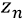as the result of the operation. Results of the multiplication are shown in Fig. 5, and the division in Fig. 6

Ex.: Shown in Figure 5.

Multiplication and division:

Module and conjugate: GeoGebra has predefined functions and commands to find the module and the conjugate of a complex number. The module is obtained with the function abs (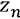) or with the command longitude [], and the conjugate with the function conjugated^ y) or with the command Refleja[EjeX].

To find the module, we enter the following in the input bar:

To find the conjugate, we enter the following in the input bar:

The value of a corresponds to the module of the number, and z1' corresponds to the conjugate (Fig. 7).

Roots of a complex number: In order to find the root of a complex number in GeoGebra, it is necessary to download an application that is available in the official GeoGebra web page (raices.ggb). After downloading the file, we opened GeoGebra, which comes with a predetermined data in the algebraic view. All values can be modified, but to obtain the result we should simply modify the complex number "A" and the value of "n" (Fig. 8).

Ex.:

Find the roots for n=4 y n=7

For n=4:

Polar form of a complex number: To represent a complex number of polar form (Fig. 9), it is necessary to download an application called complex number polar and trigonometric, which is found in the official webpage of GeoGebra. Once the .ggb file has been downloaded it can be opened in GeoGebra. The program comes with a predetermined data in the algebraic view. All the values can be modified, although to obtain the result, we simply modify the complex number.

Ex.:

Find the polar representation of:

IV. DISCUSSION

MATLAB and GeoGebra are powerful tools with their own and differentiated qualities. The fact that MATLAB uses its own programming language allows it to evolve regarding the way it treats the operations with complex numbers and some other referring subjects. To increase the observance of the virtues that this software tool can offer, it will be possible to develop a small application that performs the same operations shown in this paper automatically with the MATLAB language, in order to improve the graphical properties of the representation of each operating result, resembling more to those shown by GeoGebra, being more user friendly.

V. CONCLUSIONS

Teaching complex numbers can be simplified in some way by using graphical tools that allow anyone to retain the information they want to learn more easily. MATLAB is a very powerful mathematical software that is more oriented toward code operation using its own programming language. GeoGebra is geared more toward usability and a graphical environment. The two tools have special characteristics that make them suitable for teaching complex numbers; all depends on the appropriate choice, depending on the context in which the teaching is taking place.

ACKNOWLEDGMENTS

The authors acknowledge Universidad Distrital Francisco José de Caldas, in particular, the research group TRHISCUD (Treatment of Clinical Histories -Universidad Distrital-) of the Engineering Faculty.

REFERENCES

 G. T. Bagni, "La introducción de la historia de las matemáticas en la enseñanza de los números complejos. Una investigación experimental desempeñada en la educación media superior," Revista Latinoamericana de Investigación en Matemática Educativa, vol. 4 (1), pp. 45-61, 2001. [ Links ]

 N. Nesbitt, and G. Bright, "Elementary preservice teacher's changing beliefs and instructional use of children's mathematical thinking," Journal for research mathematics education, vol. 30 (1), pp. 99-100, 1999. [ Links ]

 M. Flores, and E. Montoya, "Artefacto y espacio de trabajo matemático en la multiplicación de números complejos," Educación matemática, vol. 28 (2), pp. 85-117, Aug. 2016. DOI: https://doi.org/10.24844/EM2802.04. [ Links ]

 E. Millán-Rojas, A. Gallego-Torres, and D. Chico-Vargas, "Simulation of a grid network with virtual machines to create a learning environment of high performance computing," Revista Facultad de Ingeniería, vol. 25(41), pp. 85-92, Jan. 2016. DOI: https://doi.org/10.19053/01211129.4140. [ Links ]

 M. Hohenwarter, J. Hohewarter, J. Kreis, and Z. Lavicza, "Teaching an learning calculus with Free Dynamics Mathematics Software Geogrebra," In 11 th International congress on Mathematical education, Monterrey; Nuevo León; México, 2008. [ Links ]

 A. D. Araujo, "Creencias acerca de la enseñanza de los números complejos," Master Thesis, Universidad del Zulia, Zulia, Venezuela, 2007. [ Links ]

 K. Choi, and H. Liu , "Matlab and Simulink Basics," Problem-Based Learning in Communication Systems Using MATLAB and Simulink, p. 400, 2016. DOI: https://doi.org/10.1002/9781119060239.ch1. [ Links ]

 G. Karakok, H. Soto-Jhonson, and A. Dyben, "Conception of various forms of complex numbers," CrossMark, Springer Science, 2014. [ Links ]

 S. Parra, "Reflexiones en torno a los modelos de formación docente dominantes y alternativos," Revista Omnia, vol. 6 (1), pp. 111-121, 2001. [ Links ]

 G. Aghekyan, "How to teach complex numbers applying the Geogebra software," Doctoral Thesis, Russian-Armenian University, Yerevan, Armenia, 2014. [ Links ]

 F. G. Perez, "Los modelos didácticos como instrumento de análisis y de intervención en la realidad educativa," Revista bibliografica de Geografía y Ciencias Sociales, vol. 207, Feb. 2000. [ Links ]

 J. I. Lopéz-Ruiz, "Abriendo puertas. Los Estudios de Casos desde un enfoque innovador y formativo," Investigación en la escuela, vol. 41, pp. 103-111, 2000. [ Links ]

Cómo citar este artículo: Acosta-López, B. Esguerra-Prieto, and N. González-Garzón, "Some mathematical software tools for an introduction to teaching of complex numbers" Rev. Fac. Ing., vol. 27 (48), pp. 79-89, May. 2018.

AUTHORS' CONTRIBUTIONS Alberto Acosta was the supervisor of the study. Brayan Esguerra and Natalia González conducted the research, collected and analyzed the documents referred in this article, and contributed to the writing of the manuscript. All the authors read and approved the final document.

Received: January 12, 2018; Accepted: April 17, 2018This is an open-access article distributed under the terms of the Creative Commons Attribution License